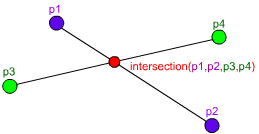## Line-Line Intersection in AS3I found a bug in my old Line-Line Intersection in C++ post, and after I fixed it I thought it would be a good idea to port it to AS3:

```function intersection(p1:Point, p2:Point, p3:Point, p4:Point):Point {
var x1:Number = p1.x, x2:Number = p2.x, x3:Number = p3.x, x4:Number = p4.x;
var y1:Number = p1.y, y2:Number = p2.y, y3:Number = p3.y, y4:Number = p4.y;
var z1:Number= (x1 -x2), z2:Number = (x3 - x4), z3:Number = (y1 - y2), z4:Number = (y3 - y4);
var d:Number = z1 * z4 - z3 * z2;

// If d is zero, there is no intersection
if (d == 0) return null;

// Get the x and y
var pre:Number = (x1*y2 - y1*x2), post:Number = (x3*y4 - y3*x4);
var x:Number = ( pre * z2 - z1 * post ) / d;
var y:Number = ( pre * z4 - z3 * post ) / d;

// Check if the x and y coordinates are within both lines
if ( x < Math.min(x1, x2) || x > Math.max(x1, x2) ||
x < Math.min(x3, x4) || x > Math.max(x3, x4) ) return null;
if ( y < Math.min(y1, y2) || y > Math.max(y1, y2) ||
y < Math.min(y3, y4) || y > Math.max(y3, y4) ) return null;

// Return the point of intersection
return new Point(x, y);
}```

You can try it here:

### 8 Responses to “Line-Line Intersection in AS3”

1.Adam Says:

Before using this for something like collision detection in a game, you will want to expand your analysis for the cases where your determinant is zero. A zero determinant (d==0) tells you there is no UNIQUE point of intersection between the lines passing through your points. The lines are either parallel or collinear. But… your function is not a line-line intersection function, it’s really a line segment intersection function, so there are more cases to breakdown when d==0:

You could have a valid intersection if the line segments are collinear and share a point. Consider the simple case where all 4 points are on the same line, and the segments share an endpoint. Your function will return null, but it really should return the intersection point (the common endpoint). Ditto for the completely degenerate case where p1==p2==p3==p4.

In the case where your segments are collinear and overlap by more than an endpoint, the most correct thing to do would be to return the segment that represents the intersection. This will require a more general return type (a segment type, i.e. two points).

2.john Says:

over 1 1/2 years… THANKS! this is a really fast function, It out performs Keith Hairs version which i was currently using.

3.Robbe Says:

Thanks! This will really come in handy.
(and also thanks john for pointing out the performance thingy, because I was about to go and test it myself :o)

4.Lucas Says:

I create framework with this code, and i have problem to specific coors:

var r:GRectangle = new GRectangle(475.5, 468.05, 475.5 + 52.39999999999998, 468.05 + 57.099999999999966);
var pA:GPoint = new GPoint(183, 504);
var pB:GPoint = new GPoint(883, 504);
trace(r.intersectsLinePoints(pA, pB)); // return FALSE

5.Lucas Says:

trace(new GLine(475.5, 468.05, 475.5, 468.05 + 57.099999999999966).intersectsLinePoints(pA, pB)); // this return null

6.Lucas Says:

if you use int instead of number works well =)

7.Michael Says:

// this will give null
var p1= new Point(x=343.4729858398438, y=351.9473937988281);
var p2= new Point(x=628.6473937988281, y=351.9473937988281);
var p3= new Point(x=486.06018981933596, y=494.5345977783203);
var p4= new Point(x=486.06018981933596, y=209.36018981933594);
trace (intersection(p1,p2,p3,p4));

// but this will draw two intersecting lines
graphics.lineStyle(1);
graphics.moveTo(p1.x,p1.y);
graphics.lineTo(p2.x,p2.y);
graphics.moveTo(p3.x,p3.y);
graphics.lineTo(p4.x,p4.y);

8.Michael Says:

Just wanted to add that i have tried Keith Hairs code and it seems to work much better:
http://keith-hair.net/blog/2008/08/04/find-intersection-point-of-two-lines-in-as3/